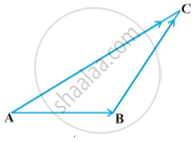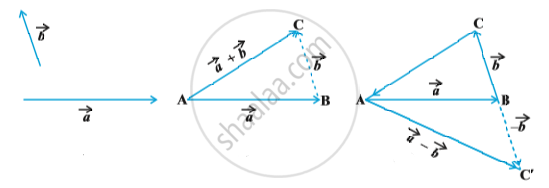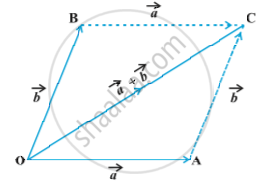#### notes

A vector  simply means the displacement from a point A to the point B. Now consider a situation that a girl moves from A to B and then from B to C in folloing fig.The net displacement made by the girl from point A to the point C, is given by the vector vec (AC)  and expressed as
vec (AC) = vec (AB) + vec (BC)
This is known as the triangle law of vector addition.
There are two vectors vec a and vec b in following  first fig. then to add them, they are positioned so that the initial point of one coincides with the terminal point of the other in following second fig.In above second fig. we have shifted vector vec b without changing its magnitude and direction, so that it’s initial point coincides with the terminal point of vec a. Then the vector vec a +vec b ,  represented by the third side AC of the triangle ABC, gives us the sum (or resultant) of the vectors vec a and vec b i.e. in triangle ABC in above fig .
vec (AB) + vec (BC) = vec (AC)
Now again, since  vec (AC) = - vec (CA), from the above equation, we have
vec (AB) + vec (BC) + vec (CA) = vec ("AA") =  vec 0
This means that when the sides of a triangle are taken in order, it leads to zero resultant as the initial and terminal points get coincided in above third fig.
Now, construct a vector vec (BC') so that its magnitude is same as the vector vec (BC),  but the direction opposite to that of it in above third fig.
vec (BC)' = - vec (BC)
Then, on applying triangle law from the Fig.
vec (AC') = vec (AB) + vec (BC') = vec (AB) +  vec ((-BC)) = vec a - vec b
The vector  vec (AC') is said to represent the difference of vec a - vec b.
If we have two vectors vec a and vec b represented by the two adjacent sides of a parallelogram in magnitude and direction in following fig.then their sum vec a + vec b is represented in magnitude and direction by the diagonal of the parallelogram through their common point. This is known as the parallelogram law of vector addition.

If you would like to contribute notes or other learning material, please submit them using the button below.

#### Video Tutorials

We have provided more than 1 series of video tutorials for some topics to help you get a better understanding of the topic.

Series 1

Series 2

### Shaalaa.com

Triangle Law of Addition Examples [00:34:27]
S
0%